Clutch Prep is now a part of Pearson
Ch. 5 - ChiralityWorksheetSee all chapters

# Racemic Mixture

See all sections
Sections
Constitutional Isomers vs. Stereoisomers
Chirality
Test 1: Plane of Symmetry
Test 2: Stereocenter Test
R and S Configuration
Enantiomers vs. Diastereomers
Atropisomers
Meso Compound
Test 3: Disubstituted Cycloalkanes
What is the Relationship Between Isomers?
Fischer Projection
R and S of Fischer Projections
Optical Activity
Enantiomeric Excess
Calculations with Enantiomeric Percentages
Non-Carbon Chiral Centers
Racemic Mixture
AchiralJohnny Betancourt

A racemic mixture represents having an equal mixture, or a perfect 1:1 ratio of enantiomers. They do not exhibit an optical activity and can be seen when performing reactions in which a chiral molecule is generated from an achiral one as in the SN1 mechanism.

## The Origin of the Word “Racemic”

The term racemic mixture, or racemate dates back to the 1800’s with Louis Pasteur and his colleague Jean Baptiste Biot. They did their work with the compound tartaric acid and its salt, tartrate, to show that a racemic mixture of these compounds did not rotate plane-polarized light, or were optically inactive.

*Note: you may also hear the term racemic acid used in place of tartaric acid to represent both forms.

Due to Pasteur’s discovery, we have a new understanding of chirality and stereochemistry.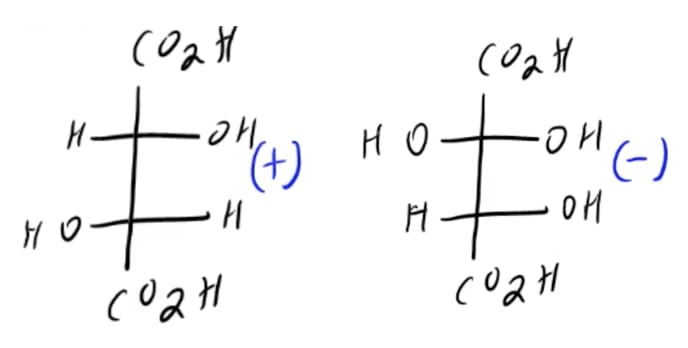These compounds seen above are (+) and (–) tartaric acid and are dicarboxylic acids in nature. Remember, CO2H is abbreviated to represent a carboxylic acid.

When we talk about tartaric acid, we will use the words dextrorotary and levorotary to describe the orientation that the compound rotates plane-polarized light. For now, just know that the (+) and (–) you see relate to these terms (more on this later).

Dextrorotary (+) and levorotary (-) are different than saying R and S! They may seem to overlap in this scenario but that is not a finite rule.

As we we continue to talk about racemic mixtures, we will use Biot’s technique of optical activity and Pasteur’s experimentation to learn more.

## Optical Activity

Optical activity is a special feature of chiral molecules. Below, we will discuss how racemic mixtures play a role, learn about how optical activity is measured, and define key terms that you may come across that can seem intimidating at first.

In addition, we will also briefly mention a few formulas along the way that you should keep handy when doing optical activity problems.

As we begin, let’s focus on what plane-polarized light really means. This term describes one of the steps that a polarimeter helps accomplish.

See, a polarimeter is what we use to measure how much rotation occurs when light passes through a chiral molecule.

First, there is a light source of some type, usually a light bulb which is our source of multidirectional light. From this light is shot through a polarizer which serves as our lens. This will filter the light and make sure it goes in one direction.

From this, we are able to get light that is plane-polarized (notice the single arrow) which means the vibration is occurring in a single plane.

After, we have the functional part of the polarimeter which can be seen in the image below as the barrel with consecutive double-headed arrows. This tube or barrel, is what carries a chiral molecule’s concentration or a mixture of molecules.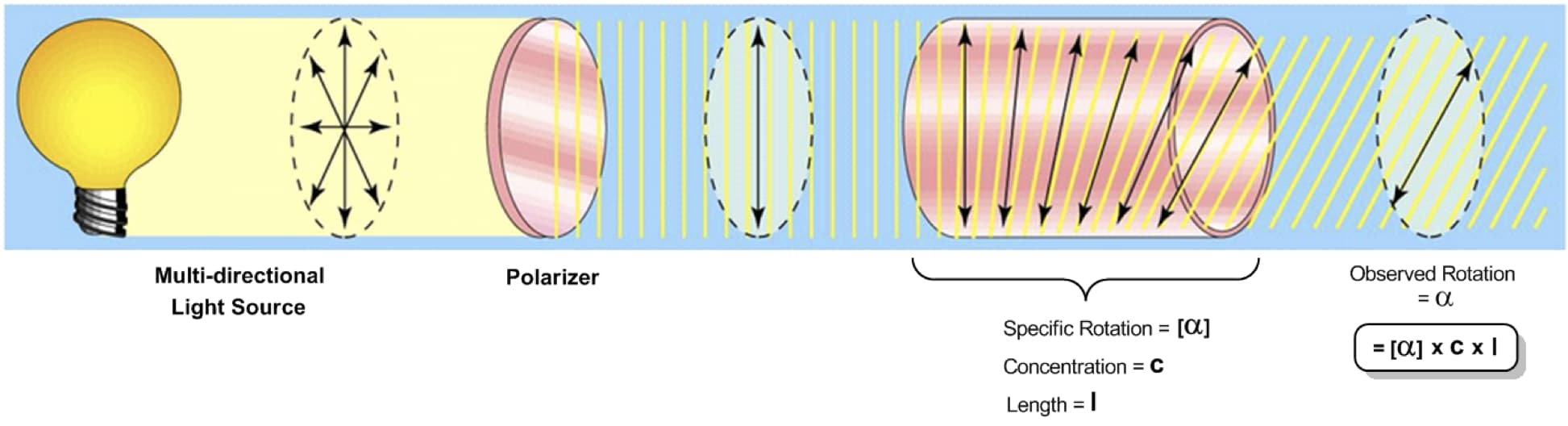As light passes through it rotates the light so that it changes its angle. This phenomon was discovered a long time ago as we mentioned above with (+) and (-) tartaric acid.

Now, every molecule has a specific rotation which has the symbol [α],

• This is basically a random number that is the amount of rotation we get with 100% of that molecule or enantiomer present. It can be positive or negative.

We also have other variables that we will use such as concentration (c), and length (l). If we combine these 3 terms we get the observed rotation (α) which has no brackets.

All these factors will play a role as to how much rotation is expected or observed, as the term implies. Racemic mixtures have an observed rotation of 0. Try to come up with a reason as to why this is the case by looking at the image below, as we dive deeper into this understanding.

Here is a mathematical explanation (scroll down to the 3rd video) as to why, however logically it comes down to the fact that opposite enantiomers have opposite rotations.

Enantiomers are a type of stereoisomers that are mirror images of each other, chiral and non-superimposable, kind of like our hands. Below we see that 2 opposite enantiomers, R and S, which are formed in a 50/50 mixture are termed racemic.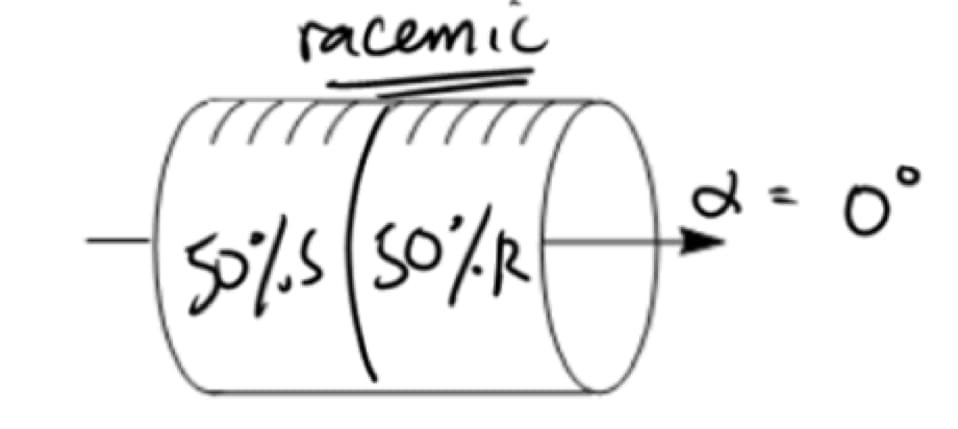Since enantiomers formed in a racemic mixture have opposite rotations, their values cancel each other out and we see no optical activity for these molecules.

When we think about rotation, there are 2 terms that you may hear repeatedly which are dextrorotary and levorotatory. These terms, as mentioned previously have nothing to do with the chirality of the molecule (i.e. dextrorotary does not mean R per se). However, here is what you need to know:

• Clockwise rotation = dextrorotary (d) or +
• Counterclockwise rotation = levorotatory (l) or –

Let’s let that information sink in for a little, before we move onto what enantiomeric excess means in a bit when we learn to solve problems.

## Stereoisomers & Chirality

Remember, chiral molecules are able to rotate plane-polarized light. What’s a chiral molecule? Well, we will look at some along with explaining in more detail what enantiomers are, how R and S is determined, and how a racemic mixture looks.

Stereoisomers and chirality were some of the key advancements made by Louis Pasteur. So let’s see how and why these terms matter.

If you recall, a stereoisomer is when two compounds have the same molecular formula, the same connectivity, however not the same shape. They actually have a different arrangement of their atoms so they are not identical.

Types of stereoisomers include enantiomers and diastereomers. Now, chiral is the term we use in organic chemistry to describe objects whose mirror image is different from the original.

The mirror image of a chiral molecule can also be called the enantiomer. Sometimes enantiomers are formed in an equal 1:1 ratio and we have a racemic mixture, but sometimes they are not formed in a perfect 1:1 ratio and we call this scalemic.

Here is an example of two compounds who are mirror images of each other, that is they are enantiomers.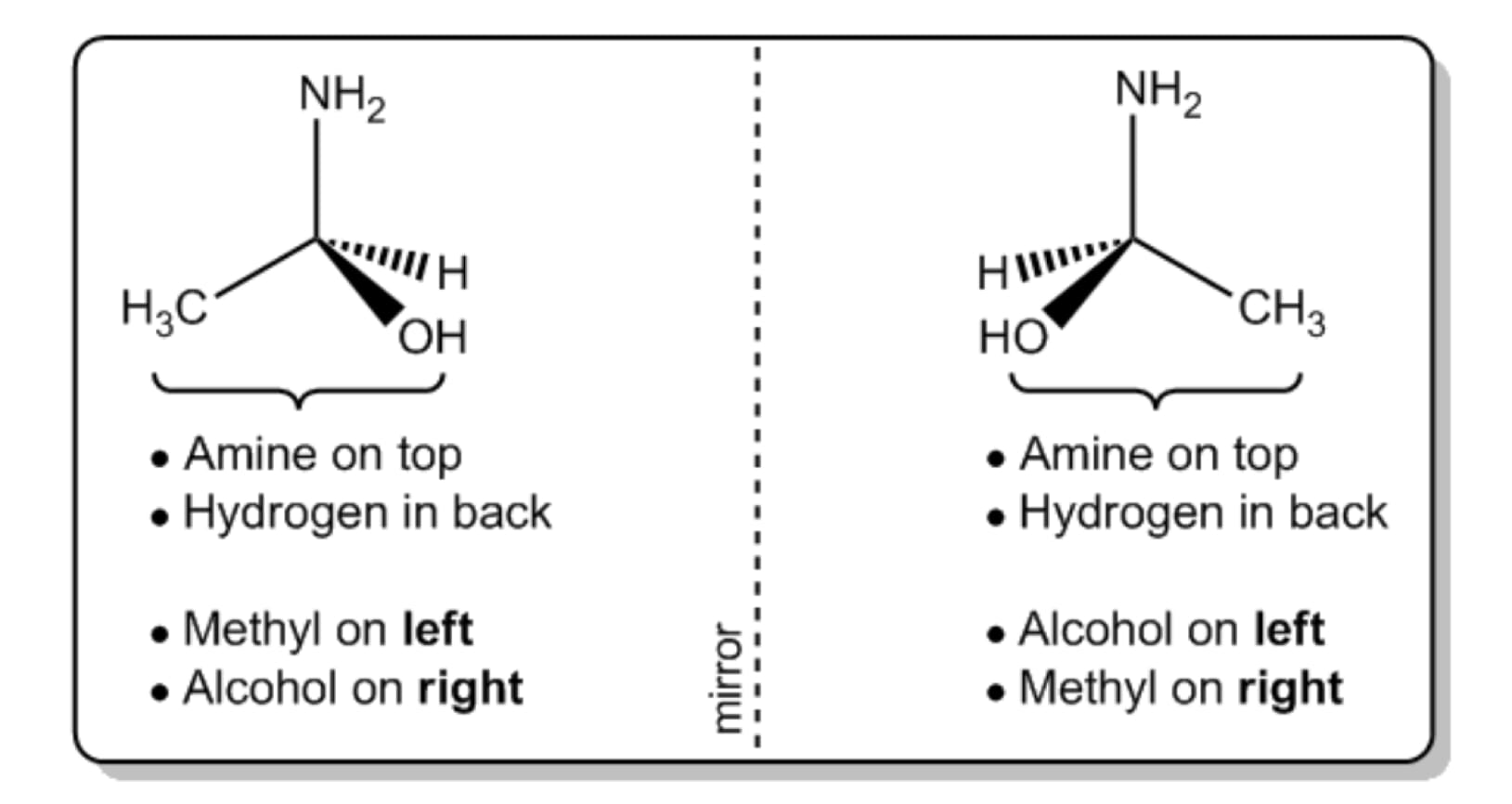Another criteria that these compounds must meet is that they cannot have an internal line of symmetry. If they do, they will be achiral.

This scenario occurs with compounds called meso compounds. Meso compounds happen when we have two (or sometimes more) symmetrical chiral centers cancelling each other out to yield 2 enantiomers that are identical.

For example: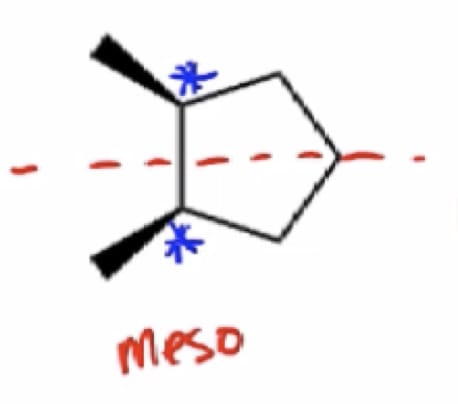This compound has an internal line of symmetry with multiple chiral centers. Also, if hard to visualize we can look for opposite configurations of the chiral center (if one is R, the other must be S).

Meso compounds uniquely have no optical activity because they are achiral. This is in contrast to racemic mixtures which have an optical activity of 0 because they perfectly cancel each other out.

## Reactions Involving a Racemic Mixture

One of the most common reactions where a racemic mixture plays a key role is in nucleophilic substitution, or SN1 reactions.

The SN1 mechanism is similar to SN2 in that you get a substitution product, but the path to get there is completely different.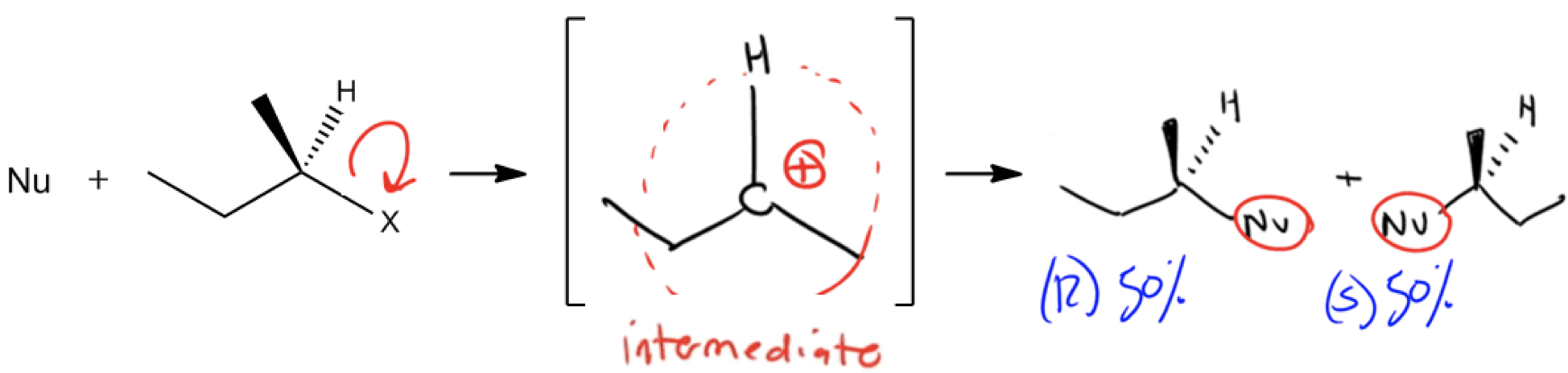Seen above is an overview of the mechanism, in which a weak nucleophile and a highly substituted leaving group are used. After the “X” leaves we form a carbocation intermediate which is attacked by a nucleophile from either the front or the back.

This step produces two products which can be formed in equal amounts of the R enantiomer or the S enantiomer if forming a new chiral center. What term can we use to describe them?

## Racemic.

Notice above that the R enantiomer and the S enantiomer each represent 50% of the product formed. This means we need to draw both products.

Now, hopefully you can see how this relates back to racemic mixtures and optical activity. Any time you hear the word racemic mixture you can relate it back to the enantiomers above. For a comparison, let’s briefly mention what we would say if one enantiomer (R or S) was formed on its own or if one enantiomer was formed in excess (i.e. 60/40).

When one enantiomer only forms we call that enantiomerically pure or a pure mixture.

When there is not a 1:1 ratio, or not a pure mixture, but somewhere in between, that is an example of a scalemic mixture or there is an enantiomeric excess. One enantiomer here would be enantioenriched.

Below, we will get into some equations that will help us solve for the different variables we mentioned above such as specific and observed rotation, as well as the enantiomeric excess.

We will also learn how to solve for enantiomeric percentages to figure out the exact amount of each enantiomer in solution.

## Sample Problems & Formulas

(I apologize in advance for the use of formulas & math in organic chemistry)

This is one of the few times in organic chemistry where reaction mechanisms and drawing organic compounds is not used.

### A. The “Formulas”

The first formula you may come across is the formula for observed rotation that we mentioned above. Here, α = the observed rotation, [α] = specific rotation, c = concentration and l = length.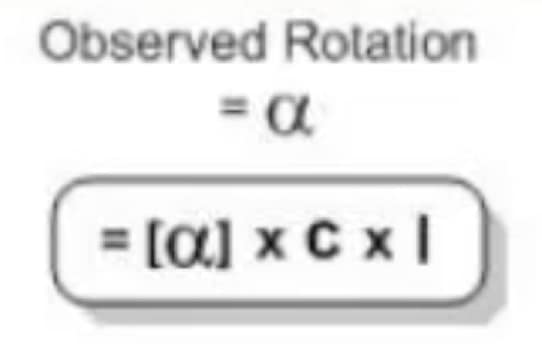You may find yourselves using this formula whenever units are mentioned such as grams, liters, or meters. Concentration here will be represented as “g/ml” and length will be measured in decimeters or “dm”. Therefore, be mindful of your unit conversions for these problems.

A common derivative of this formula that you may see is when you are being asked to solve for the specific rotation.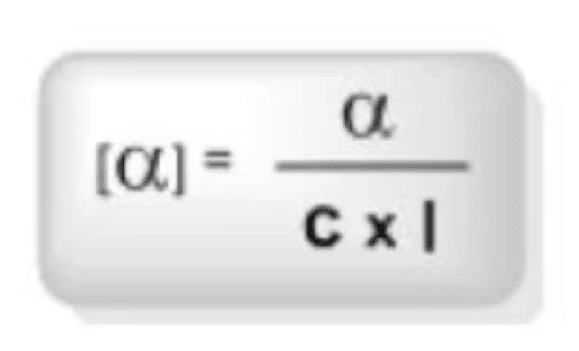The next set of formulas you may see are for “ee”, which stands for enantiomeric excess and a formula for observed rotation where specific rotation and “ee” are related.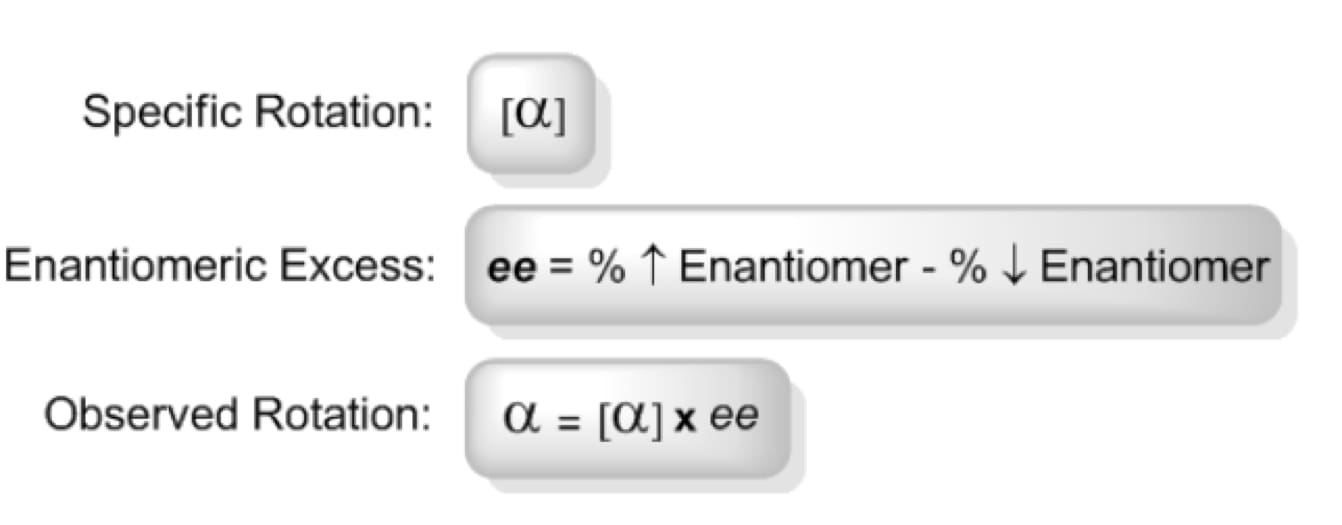These formulas can be seen used to calculate for enantiomeric excess (ee) using the two percentages of enantiomers, which can then be multiplied by the specific rotation to give us the observed rotation for a mixture.

We will go over an example of this in part B.

The last set of formulas you will need to know will be used primarily for part C, however 2 out of the 3 you should already know because they are derivatives of the ones above.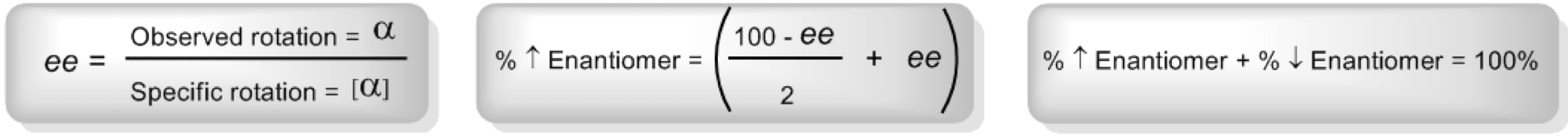The one of the left is the shuffled around version of the observed rotation formula, while the one on the right is the enantiomeric excess formula in different terms.

The middle formula is a new one however. It says that if we calculate the enantiomeric excess using the formula on the left, we can find out how much of the higher percentage enantiomer we have and therefore also the amount of the lower percentage enantiomer.

These formulas will make much more sense once we a apply them, so let’s get started

## B. Solving for Specific and Observed Rotation

Example 1: Calculate the ee and observed rotation for the following chiral mixture where S-enantiomer has [α] = +20.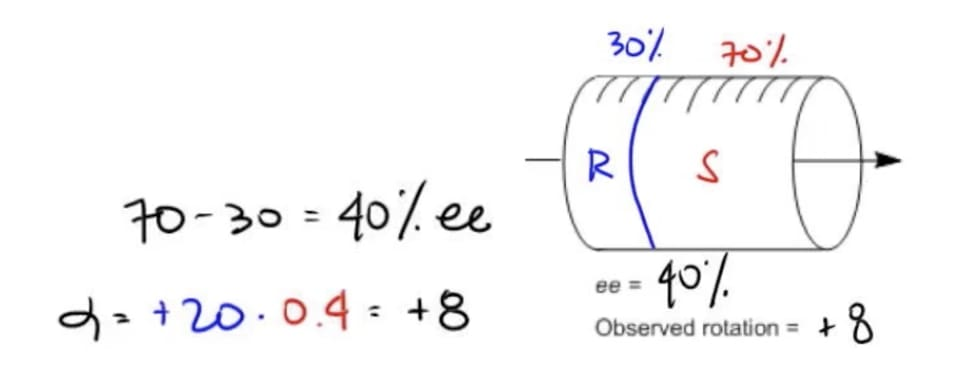In this first problem we are asked to find the enantiomeric excess and the observed roation. Therefore, we can go back to our formulas to show that the “ee” is equal to the percent of the higher enantiomer minus the lower enantiomer. (%↑ enantiomer - % ↓ enantiomer).

70% is the S enantiomer, while 30% is the R enantiomer (seen above) therfore we get an “ee” of 40%. Now, next we can plug this number in after we convert it to a decimal of .4 and use our specific rotation [α] value given in the question to solve the rest.

The “ee” of 40% means that this is the optically active part of the molecule while everything to the left in green is racemic and not optically active. The S enantiomer would love to rotate +20 but the only part that’s available is 40% so it rotates +8, which is our observed rotation.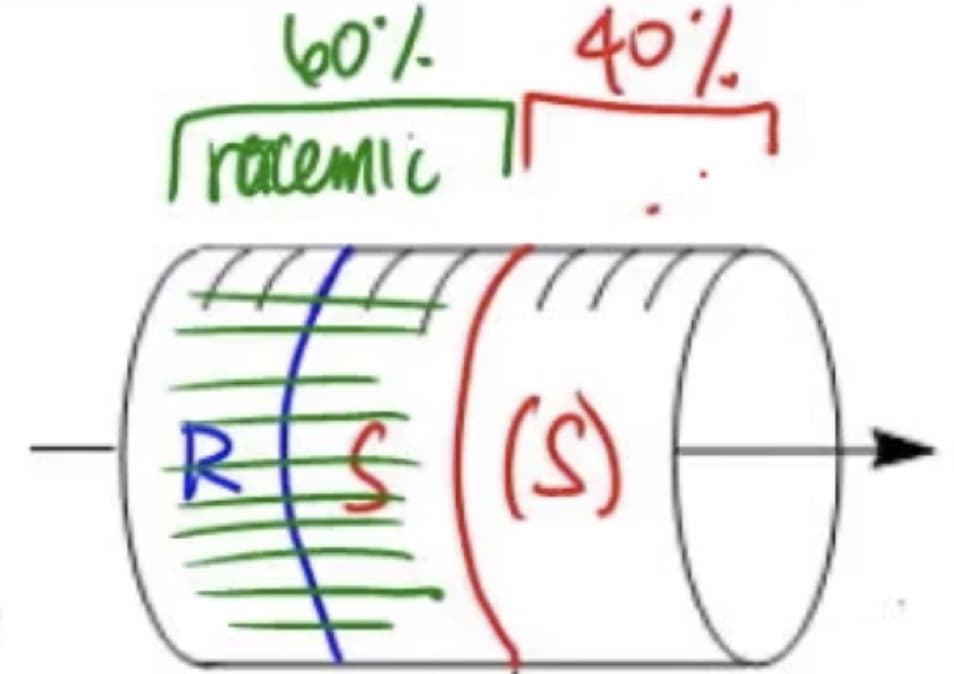Remember, specific rotation is the rotation that 100% pure enantiomers produce. Also don’t forget that the opposite enantiomer would have the opposite specific rotation.

I hope that first problem wasn’t too bad. Let’s take a look at the next one and see how you do!

Example 2: Calculate the observed rotation of a chiral mixture that contains 65% (S)-stereoisomer where the [α] of pure (S)-stereoisomer = -118.

Here, we will also be solving for the observed rotation but now given the percentage of one enantiomer and the specific rotation.

We can use the same formula we used in the previous problem which is α = [α] x ee. We were given the specific rotation [α] already, so that means we just need to find the ee.

To find the ee, we will simply use the knowledge that the percentage of both our enantiomers must equal a whole, or 100. That means we must subtract 65, which is our percentage of our S enantiomer, from 100 to give us the % of the lower enantiomer, R.

Once we find this we can use our formula for calculating ee, and then plug that value into our equation using ee = 30%, and [α] = -118.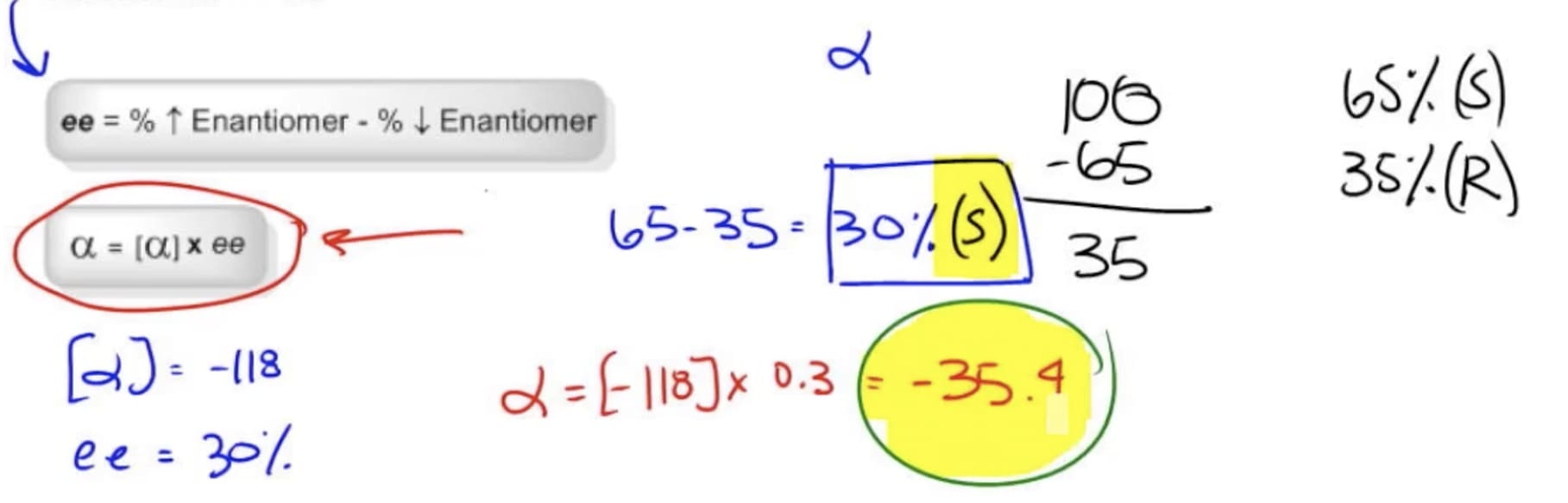Pretty easy, right? However, I want to fill you in on 1 piece of information that may complicate this problem.

If the R enantiomer was to be in excess because it was the higher percentage enantiomer, then we would have to flip the sign from – to +. Why? Because we know that opposite enantiomers have opposite rotations.

Make sense? I hope so! Now, let’s move on to our final problem which will be a bit more challenging.

### C. Solving for Enantiomeric Percentages

Example 3: The [α] of pure S-epinephrine is +50°. Calculate the ee of a solution with an observed value of -40°. Calculate percent of each enantiomer. Then sketch the approximate mixture in our sample polarimeter tube.

In this last example, we are trying to solve for the percent of each enantiomer. How are we going to do that? Well, we will be employing a new formula.

That formula is one of the last three that we talked about in the “formula section which is used to calculate the percentage of the higher enantiomer using the ee.

So to start I always like to write down what we were given. In the question it states the specific rotation, and the observed rotation. Can we find the ee with these values?...

• Yes!

In order to do so we simply can use the derivate of the formula: α = [α] x ee

That means we will say that: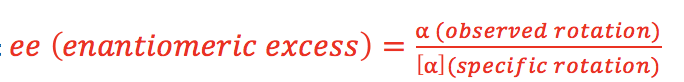That gives us a value of -80% as you can see below.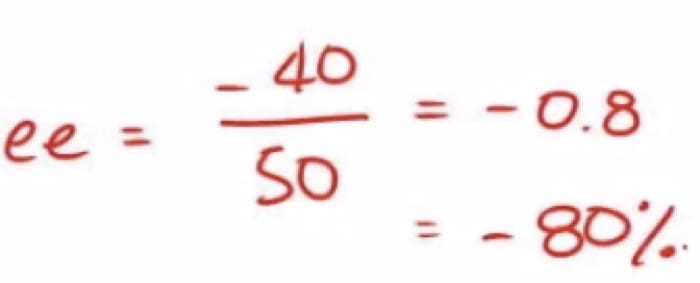However, since this value is negative this means we have an excess of the other enantiomer from the problem. This means the 80% is for the R enantiomer, not the S.

After this, the rest is smooth sailing. We simply need to find out the % of both enantiomers.

One way to solve the problem is through logic, not formulas. If we know 80% excess R, that means the rest must be racemic. That is, 10% R, 10% S.

Or we can use the formula which states that: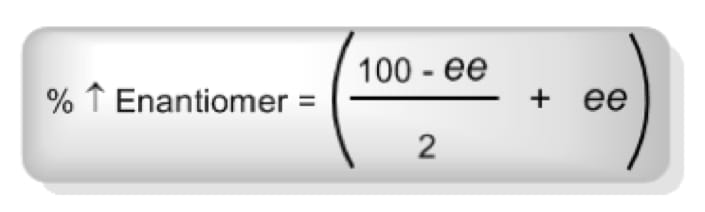If we do this mathematically we will come to the conclusion that we have 90% of the R enantiomer, and 10% of the S enantiomer, since both must add up to 100%.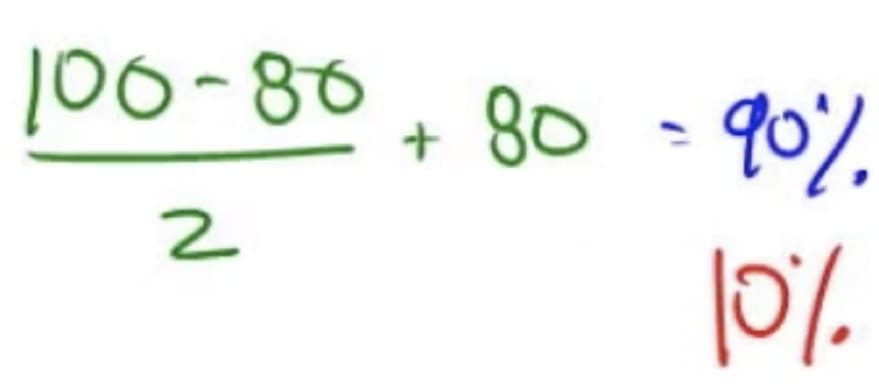Lastly, we were asked to sketch the approximate mixture in our sample tube. To see what that looks like you can check your work with the image below. Good job for making it through that problem!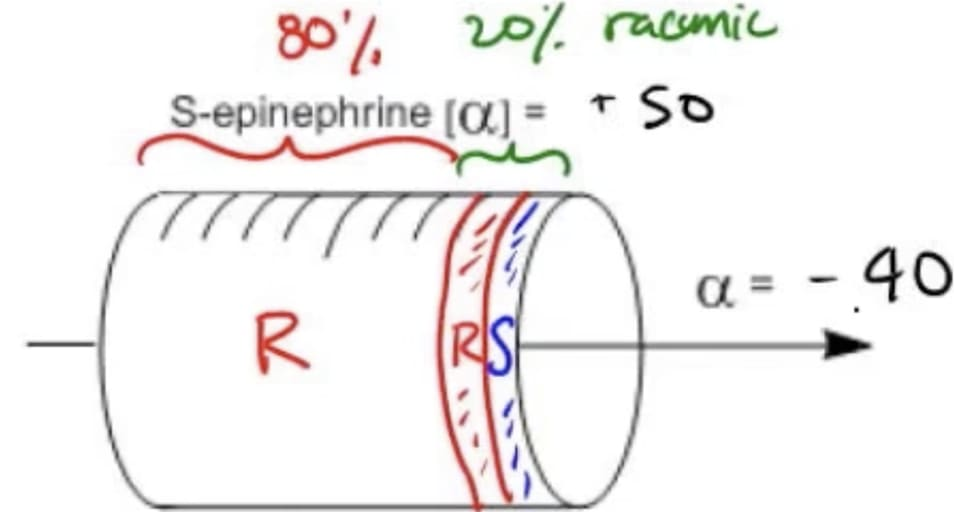Would (+) and (-) tartaric acid have the same physical properties? (i.e. melting point)

• Yes, because they are enantiomers of each other and therefore have the same physical properties, besides rotating plane-polarized light opposite directions. Note that any other mixture such as a meso or racemic form of tartaric acid would not have the same physical properties.

Is dextrorotary R or S?

• No, dextrorotary has to do with a molecule rotating plane-polarized light clockwise, while R and S deal with the arrangement of atoms for a chiral molecule or group of atoms.

Is a meso compound optically active?

• No they are not. The reason is because meso compounds by definition are achiral. For a molecule to be optically active it must first be chiral.Johnny Betancourt

Johnny got his start tutoring Organic in 2006 when he was a Teaching Assistant. He graduated in Chemistry from FIU and finished up his UF Doctor of Pharmacy last year. He now enjoys helping thousands of students crush mechanisms, while moonlighting as a clinical pharmacist on weekends.